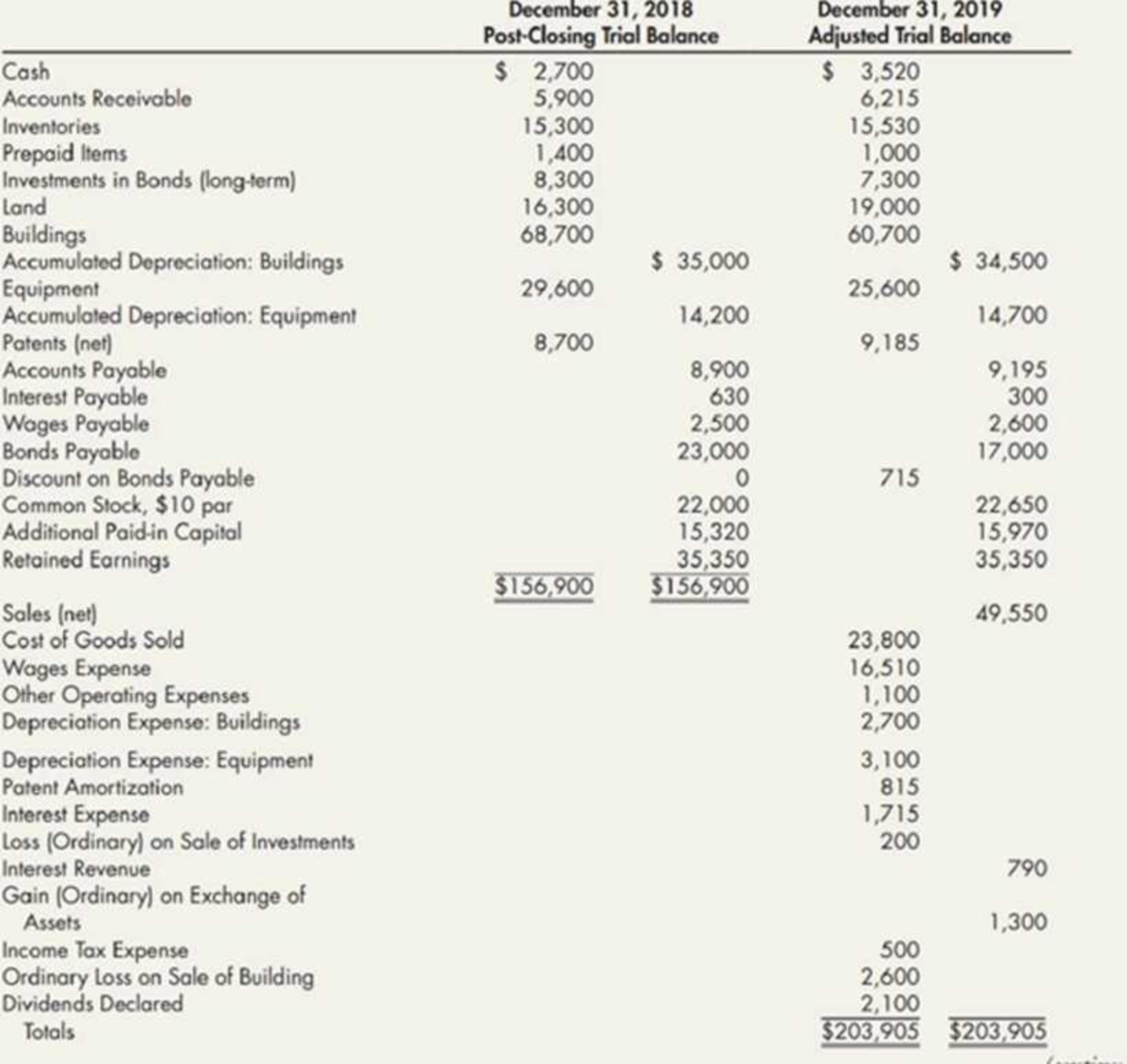Chapter 21, Problem 18P### Intermediate Accounting: Reporting...

3rd Edition
James M. Wahlen + 2 others
ISBN: 9781337788281

#### Solutions

Chapter
Section### Intermediate Accounting: Reporting...

3rd Edition
James M. Wahlen + 2 others
ISBN: 9781337788281
Textbook Problem
1 views

# Spreadsheet from Trial Balance Heinz Company’s post-closing trial balance as of December 31, 2018, and the adjusted trial balance as of December 31, 2019, are shown here:A review of the accounting records reveals the following additional information: a. Bonds payable with a face value, book value, and market value of $14,000 were retired on June 30, 2019. b. Bonds payable with a face value of$8,000 were issued at 90.25 on August 1, 2019. They mature on August 1, 2024. The company uses the straight-line method to amortize the bond discount. c. The company sold a building that had an original cost of $8,000 and a book value of$4,800. The company received $2,200 in cash for the building and recorded a loss of$2,600. d. Equipment with a cost of $4,000 and a book value of$1,400 was exchanged for an acre of land valued at $2,700. No cash was exchanged. e. Long-term investments in bonds being held to maturity with a cost of$ 1,000 were sold for $800. f. Sixty-five shares of common stock were exchanged for a patent. The common stock was selling for$20 per share at the time of the exchange. Required:Prepare a spreadsheet to support a statement of cash flows for 2019.(Appendix 21.1) Complex Spreadsheet Refer to the information for Нсіпг Company in P21-8.Required:Using the direct method for operating cash flows, prepare a spreadsheet to support a 2019 statement of cash flows.

To determine

Prepare a spreadsheet for operating cash flows using direct method for the year 2019.

Explanation

Statement of cash flows: This statement reports all the cash transactions which are responsible for inflow and outflow of cash, and result of these transactions is reported as ending balance of cash at the end of reported period. Statement of cash flows includes the changes in cash balance due to operating, investing, and financing activities.

Worksheet: A worksheet is a spreadsheet used while preparing a financial statement. It is a type of form having multiple columns and it is used in the adjustment process. The use of a worksheet is optional for any organization. A worksheet can neither be considered as a journal nor a part of the general ledger.

Prepare a spreadsheet for operating cash flows using direct method.

Table (1)

Prepare a statement of cash flows using direct method.

Table (2)

Working notes:

a) Net sales for the year 2019 are $49,550. b) Interest received for the year 2019 is$790.

c) Cost of goods sold for the year 2019 is $23,800. e) Other operating expenses paid during the year are$1,100.

f) Payment of interest during the year is $1,715. g) Income tax paid during the year is$500.

k) Calculate the changes in accounts receivable.

Change in accounts receivable = Closing balanceOpening balance=$6,215$5,900=$315 l) Calculate the change in inventories. Change in inventories = Closing balanceOpening balance=$15,530$15,300=$230

m) Calculate the change in prepaid items.

Change in prepaid items = Opening balanceclosing balance=$1,400$1,000Decrease in prepaid items=$400 n) Calculate the change in accounts payable. Change in accounts payable= Closing balanceOpening balance=$9,195$8,900=$295

o) Calculate the change in Interest payable

### Still sussing out bartleby?

Check out a sample textbook solution.

See a sample solution

#### The Solution to Your Study Problems

Bartleby provides explanations to thousands of textbook problems written by our experts, many with advanced degrees!

Get Started

#### Find more solutions based on key concepts